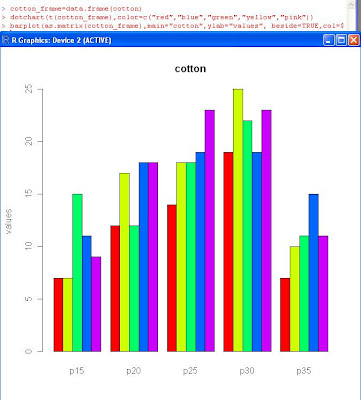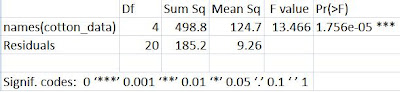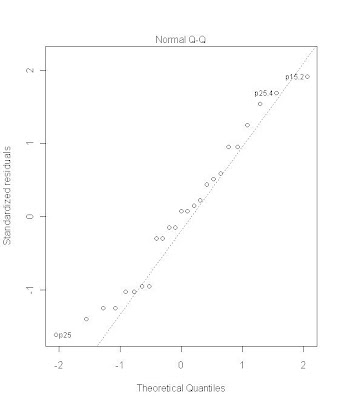# Examples using R – Analysis of Variance

Problem: A product development engineer is interested in investigating the tensile strength of a new synthetic fiber that will be used t omake cloth for men’s shirts. The engineer knows from previous experience that the strength is affected by he weight percent of cotton used in the blend of materials for the fiber. Furthermore, she suspects that increasing the cotton content will increase the strength, at least initially. she also knows that cotton content should range between about 10 and 40 percent if the final product is to have other quality characteristics that are desired. The engineer decides to test specimens at five levels of cotton weight percent: 15, 20, 20, 30 and 35 percent. she also decides to test 5 specimens at each level of cotton content.
Here we have a single factor with 5 levels and 5 replicates.The data is

> cotton
\$p15
 7 7 15 11 9
\$p20
 12 17 12 18 18
\$p25
 14 18 18 19 23
\$p30
 19 25 22 19 23
\$p35
 7 10 11 15 11
A box plot of the data isA histogram of data looks likeA multiple scatter plot can sometimes be used if corresponding values of the observations need comparison. The scatter plot for this data is as shown.Analysis of Variance:
Lets use analysis of variance in the above example to find out if all means are equal or if any mean is different.
The data needs to be transformed for aov

> c(cotton_matrix[1,],cotton_matrix[2,],cotton_matrix[3,],cotton_matrix[4,],cotton_matrix[5,])->cotton_data
> cotton_data
p15 p20 p25 p30 p35 p15 p20 p25 p30 p35 p15 p20 p25 p30 p35 p15 p20 p25 p30 p35 p15 p20 p25 p30 p35
7 12 14 19 7 7 17 18 25 10 15 12 18 22 11 11 18 19 19 15 9 18 23 23 11

Analysis of variance yields

> summary(aov(cotton_data~names(cotton_data)))from the F value we reject the null hypothesis and conclude that the means differ.

Analysis of variance uses certain assumptions and it is important to check the validity of these assumptions. The first method is to analyse the residuals for each observations. There should be no pattern in the residuals. If residuals either spread out or narrow down as time progresses then this could be an experimental error.
Here’s a plot of residuals against time (observation)Another validation is to check the nature of the residuals themselves. One way to do is to plot of curve of residuals versus the fitted values.Here again no pattern should be presentThe variance for the five sets can be compared using the Bartlett’s test
> bartlett.test(cotton_data~factor(names(cotton_data)))
Bartlett test of homogeneity of variances
data: cotton_data by factor(names(cotton_data))
Bartlett’s K-squared = 0.2801, df = 4, p-value = 0.991

The results show that the null hypothesis cannot be rejected and hence the variance of the five sets is indeed same.

We now need to do a pairwise comparison to find out which pair has a difference in mean. we use the Tukey’s test to do so.If the assumption of normality is not met then a test known is Kruskal-Wallis test may be used

> kruskal.test(cotton_data~factor(names(cotton_data)))
Kruskal-Wallis rank sum test
data: cotton_data by factor(names(cotton_data))
Kruskal-Wallis chi-squared = 18.5513, df = 4, p-value = 0.0009626